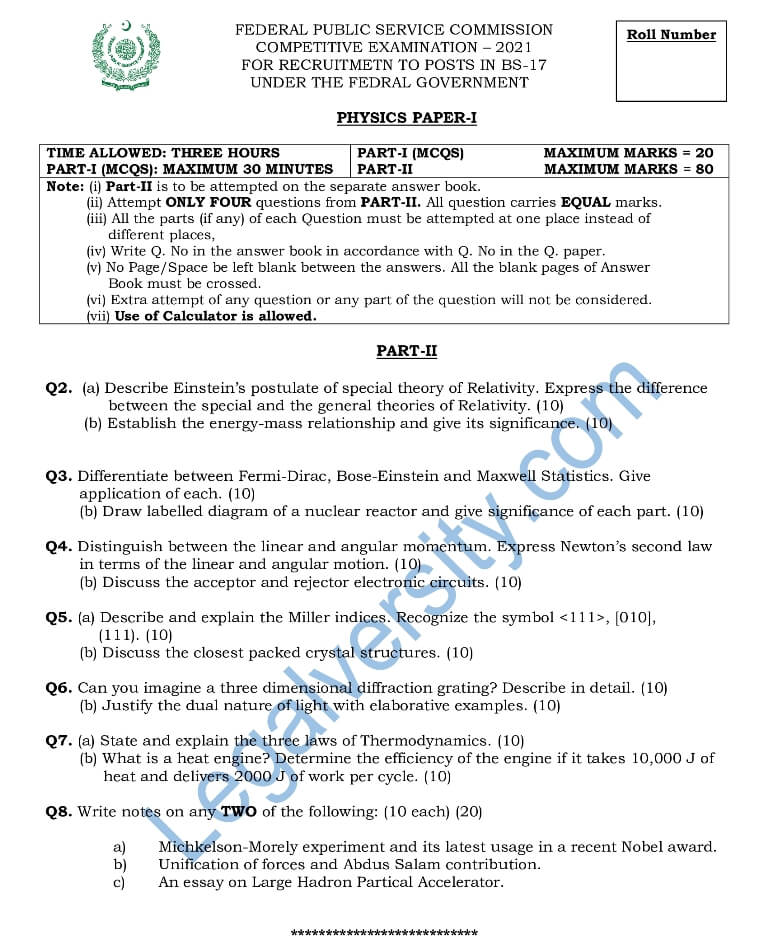# CSS Physics Paper-I 2021

In this article, you will find the CSS Physics Paper-I Paper 2021. You can view or download this CSS Physics Paper-I Paper 2021. The Physics paper-I of CSS was held on 25-02-2021.

### Get CSS Physics Paper-I Paper 2021

The following questions were given in the CSS Physics Paper-I Paper of 2021:

Q2.  (a) Describe Einstein’s postulate of the special theory of Relativity. Express the difference between the special and the general theories of Relativity.
(b) Establish the energy-mass relationship and give its significance.

Q3. Differentiate between Fermi-Dirac, Bose-Einstein, and Maxwell Statistics. Give the application of each. (10)
(b) Draw a labeled diagram of a nuclear reactor and give the significance of each part. (10)

Q4. Distinguish between the linear and angular momentum. Express Newton’s second law in terms of linear and angular motion. (10)
(b) Discuss the acceptor and rejector electronic circuits. (10)

Q5. (a) Describe and explain the Miller indices. Recognize the symbol <111>, , (111). (10)
(b) Discuss the closest packed crystal structures. (10)

Q6. Can you imagine a three-dimensional diffraction grating? Describe in detail. (10)
(b) Justify the dual nature of light with elaborative examples. (10)

Q7. (a) State and explain the three laws of Thermodynamics. (10)
(b) What is a heat engine? Determine the efficiency of the engine if it takes 10,000 J of heat and delivers 2000 J of work per cycle. (10)

Q8. Write notes on any TWO of the following: (10 each) (20)

1. Mickelson-Morely experiment and its latest usage in a recent Nobel award.
2. Unification of forces and Abdus Salam’s contribution.
3. An essay on Large Hadron Particle Accelerator.Other CSS (Compulsory) 2021 Papers:

Other CSS (Optional) 2021 Papers:

➤➤ View All CSS Papers of 2021 here

You may also like these:

Subscribe
Notify of